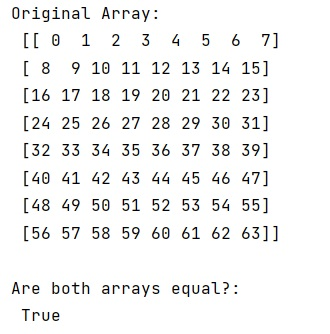# How to convert byte array back to NumPy array?

Learn, how to convert byte array back to NumPy array in Python?
Submitted by Pranit Sharma, on February 19, 2023

NumPy is an abbreviated form of Numerical Python. It is used for different types of scientific operations in python. Numpy is a vast library in python which is used for almost every kind of scientific or mathematical operation. It is itself an array which is a collection of various methods and functions for processing the arrays.

## Converting byte array back to NumPy array

Suppose that we are given a numpy array and we are converting it into a byte array. Once the array is converted to a byte array and some operation is performed, we need to convert it back into a numpy array.

In numpy, we can convert a numpy array to bytes using tobytes() function. To convert it back into a numpy array, we use numpy.frombuffer().

For this purpose, we will first create a numpy array and convert it into a byte array using tobytes() and then we will convert it back using numpy.frombuffer() to verify the result, we can use numpy.array_equal() where we can pass both the array to check if both arrays are equal or not.

Let us understand with the help of an example,

## Python code to convert byte array back to NumPy array

```# Import numpy
import numpy as np

# Creating a numpy array
arr = np.arange(8*8).reshape(8, 8)

# Display original array
print("Original Array:\n",arr,"\n")

# Converting array into byte array
by = arr.tobytes()

# Converting back the byte array into numpy array
res = np.frombuffer(by, dtype=arr.dtype)

# Checking both arrays
ans = np.array_equal(res.reshape(8, 8), arr)

# Display result
print("Are both arrays equal?:\n",ans)
```

Output: